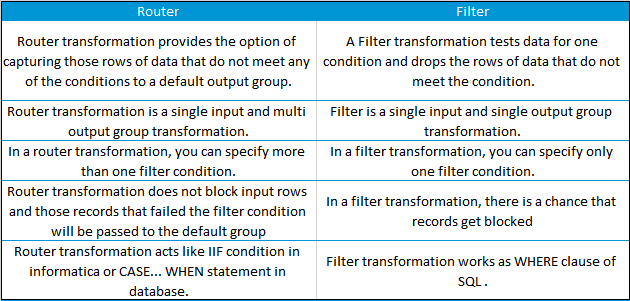# ROUTER TRANSFORMATION

1. WHAT IS THE DIFFERENCE BETWEEN ROUTER AND FILTER?
2. WHAT IS THE MINIMUM NUMBER OF GROUPS WE CAN DECLARE IN A ROUTER TRANSFORMATION?
3. SCENARIO IMPLEMENTATION 1
4. SCENARIO IMPLEMENTATION 2
5. SCENARIO IMPLEMENTATION 3

#### 90.Mention few advantages of Router transformation over Filter transformation.

Router transformation and Filter transformation are the same because both of them use a condition to test and filter the data.

However, the advantages of Router over filter transformation can be understood by the below-mentioned points.

Router Transformation:

• Allows more than one test conditions.
• Provide the ability to test the same input data on a multiple number of conditions.
• In case of mapping, input data is processed only once by the server and hence performance is improved.
• Less complex and more efficient.
• The records that fail the test condition are never blocked instead are passed on to the default group.
• Better Performance; because in mapping, the Router transformation Informatica server processes the input data only once instead of as many times, as you have conditions in Filter transformation.
• Less complexity; because we use only one Router transformation instead of multiple Filter transformations.
• Router transformation is more efficient than Filter transformation.

For E.g.:

Imagine we have 3 departments in source and want to send these records into 3 tables. To achieve this, we require only one Router transformation. In case we want to get same result with Filter transformation then we require at least 3 Filter transformations.

Similarity:

A Router and Filter transformation are almost same because both transformations allow you to use a condition to test data.

Super User(All the privileges are granted to the user).

91.What are the types of groups in router transformation?

• Input group
• Output group
• Default group

92.What is Router Transformation?

Router Transformation is used to filter the source data. You can use Router Transformation to split out a  single data source.

It is much like Filter Transformation but the only difference is that Filter Transformation uses only one transformation condition and returns the rows that do not fulfill the condition, Whereas Router Transformation uses multiple transformation conditions and returns the rows that match even a single condition.

93. What is the difference between Router and Filter?

Following differences can be note:In filter transformation the records are filtered based on the condition and rejected rows are discarded. In Router the multiple conditions are placed and the rejected rows can be assigned to a port.

94. Scenario Implementation 1
Loading Multiple Target Tables Based on Conditions- Suppose we have some serial numbers in a flat filesource. We want to load the serial numbers in two target files one containing the EVEN serial numbers and  the other file having the ODD ones.

After the Source Qualifier place a Router Transformation. Create two Groups namely EVEN and ODD, with
filter conditions as:

•  MOD(SERIAL_NO,2)=0
•  MOD(SERIAL_NO,2)=1

Then output the two groups into two flat file targets.95. Scenario Implementation 2
Suppose we have a source table and we want to load three target tables based on source rows such that first
row moves to first target table, second row in second target table, third row in third target table, fourth row
again in first target table so on and so forth. Describe your approach.

We can clearly understand that we need a Router transformation to route or filter source data to the three target tables. Now the question is what will be the filter conditions.
First of all we need an Expression Transformation where we have all the source table columns and along with that we have another i/o port say seq_num, which gets sequence numbers for each source row from the port NEXTVAL of a Sequence Generator start value 0 and increment by 1.
Now the filter condition for the three router groups will be:

•  MOD(SEQ_NUM,3)=1 connected to 1st target table
•  MOD(SEQ_NUM,3)=2 connected to 2nd target table
•  MOD(SEQ_NUM,3)=0 connected to 3rd target table96. Scenario Implementation 3
How can we distribute and load ‘n’ number of Source records equally into two target tables, so that each
have ‘n/2’ records?